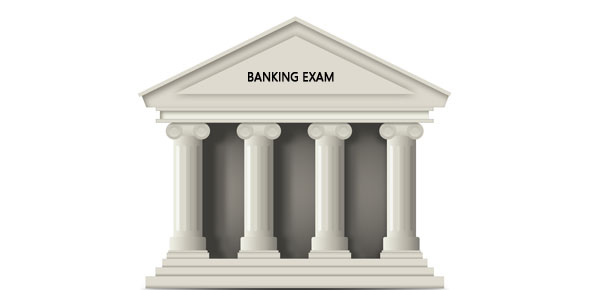# Ibps Quantitative Ability Test 1

10 Questions | Total Attempts: 66SettingsTEST DESCRIPTION Total No. Of Questions = 10 Test Duration = 15 minutes Total Marks = 40 Negative Marking = YES (1 mark will be deducted for each wrong answered question)

• 1.
A shopkeeper earns a profit of 12% on selling a book at 10% discount on the printed price. The ratio of the cost price to the printed price of the book is—
• A.

99 : 125

• B.

55 : 69

• C.

50: 61

• D.

45 : 56

• 2.
The ratio of the number of boys and girls in a school is 3 : 2. If 20% of the boys and 25% of the girls are scholarship holders, the percentage of the school students who are not scholarship holders, is—
• A.

78

• B.

80

• C.

56

• D.

70

• 3.
A does half as much work as B and C does half as much work as A and B together. If C alone can finish the work in 12 days, then together all will finish the work in—
• A.

6 days

• B.

7 days

• C.

4 days

• D.

5 days

• 4.
Taps A and B can fill a bucket in 12 minutes and 15 minutes respectively. If both are opened and A is closed after 3 minutes, how much further time would it take for B to fill the bucket?
• A.

8 mm. 5 sec

• B.

8 mm. 25 sec

• C.

7 mm. 45 sec

• D.

7 mm. 15 sec

• 5.
A train -110 m long passes a man, running at 6 kmph in the direction opposite to that of the train in 6 seconds. The speed of the train is—
• A.

54 km/hr

• B.

72 km/hr

• C.

60 km/hr

• D.

66 km/hr

• 6.
A boat takes 4 hours for travelling downstream from point A to point B and coming back to point A upstream. If the velocity of the stream is 2 kmph and the speed of the boat in still water is 4 kmph, whit is the distance between A and B?
• A.

8 km

• B.

9 km

• C.

4 km

• D.

6 km

• 7.
A man covered a-distance of 2000 km in 18 hours partly by bus at 72 kmph and partly by train at 160 kmph. The distance covered by bus is—.
• A.

860 km

• B.

640 km

• C.

1280 km

• D.

720 km

• 8.
The difference between simple interest and compound. Interest on Rs. 1200 for one year at 10% per annum, reckoned half yearly is—
• A.

Rs. 8.80

• B.

Rs. 3

• C.

Nil

• D.

Rs. 13.20

• 9.
When simplified, the product                                                                                                                                                            (2 – 1/2) (2 – 3/5) (2- 5/7)…….(2 -997/999) is equal to
• A.

5/999

• B.

1001/999

• C.

1001 /3

• D.

None of these

• 10.
The 1east perfect square number which is divisible by 3, 4, 5, 6,and 8 is—
• A.

900

• B.

1600

• C.

2500

• D.

3600

Related TopicsBack to top